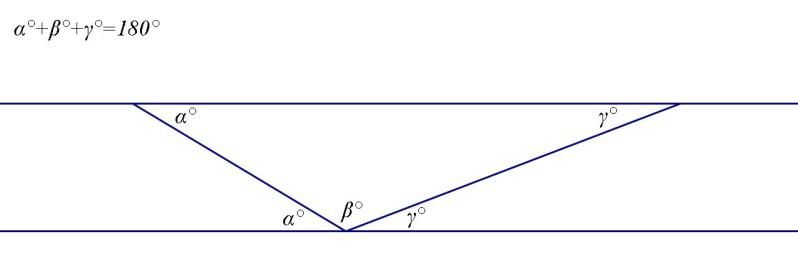# Thread: Interior Angle Sum

1. ## Interior Angle Sum

If l1 and l2 are lines are lines on the plane such that they are parallel transports along a transversal l, then they are parallel transports along any transversal.

Use the above theorem to prove that the interior angle sum of a triangle is 180.

2.The angles marked $\displaystyle \alpha^{\circ}$ are equal because they are alternate (Z) angles.

The angles marked $\displaystyle \gamma^{\circ}$ are equal because they are alternate (Z) angles.

#### Search Tags

angle, interior, sum